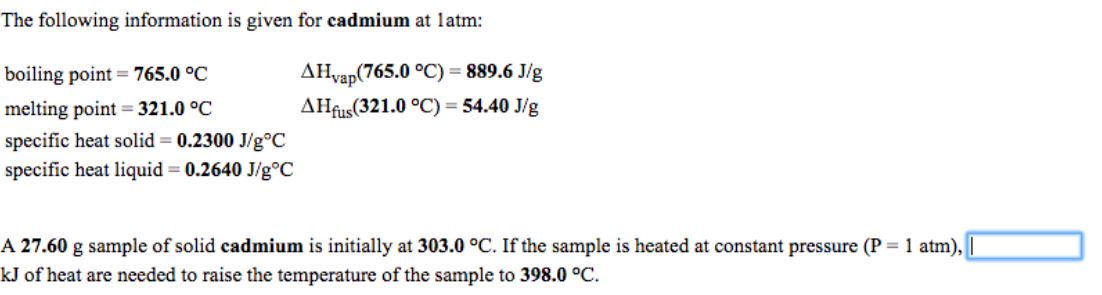# Problem: The following information is given for cadmium at 1 atm:boiling point = 765.0°CΔHvap(765.0°C) = 886.6 J/gmelting point = 321.0°CΔ Hfus(321.40°C) = 54.40 J/gspecific heat solid = 0.2300 J/g° Cspecific heat liquid = 0.2640 J/g°CA 27.60 g sample of solid cadmium is initially at 303.0°C. If the sample is heated at constant pressure (P = 1 atm), kJ of heat are needed to raise the temperature of the sample to 398.0°C.

🤓 Based on our data, we think this question is relevant for Professor Purse's class at SDSU.

###### FREE Expert Solution###### Problem Details

The following information is given for cadmium at 1 atm:

boiling point = 765.0°C
ΔHvap(765.0°C) = 886.6 J/g
melting point = 321.0°C
Δ Hfus(321.40°C) = 54.40 J/g
specific heat solid = 0.2300 J/g° C
specific heat liquid = 0.2640 J/g°C

A 27.60 g sample of solid cadmium is initially at 303.0°C. If the sample is heated at constant pressure (P = 1 atm), kJ of heat are needed to raise the temperature of the sample to 398.0°C.